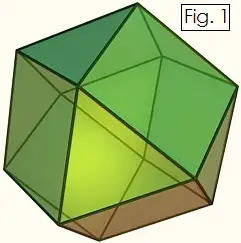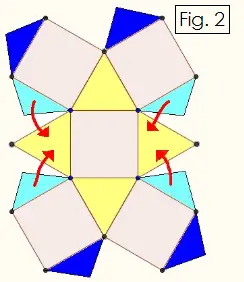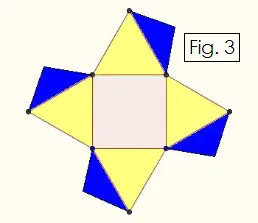# Constructing Geometric Solids:Cuboctahedron

A cuboctahedron (or cubocta) is a 14-sided solid figure in which eight of the faces are equilateral triangles and six of the faces are squares. One way to think about a cuboctahedron is that it is a cube whose eight corners have been sliced off. It is also the "dual" the rhombic dodecahedron, since the vertices of the cubocta correspond to the faces of the rhombic dodecahedron and vice versa. The name "cuboctahedron" comes from the fact that it has six square faces (cube), and eight equilateral triangle faces (octahedron). You can construct a cuboctahedron with stiff paper and glue by following the instructions and pattern below.First, look at the Figure 1 on the right to see how a cuboctahedron is built from squares and equilateral triangles. At each vertex, four faces intersect: two squares and two triangles, with square opposite square and triangle opposite triangle.Draw and cut out the shape shown in Figure 2. To draw an equilateral triangle, recall that all the interior angles are equal to 60°. Crease all of the edges upward. Glue only the light blue tabs onto the equilateral triangles as shown. The light blue tabs may overlap a little.

Next, cut out the shape shown in Figure 3 below. Crease all the edges upward. Do not glue any tabs yet. This piece fits onto the piece you made above and completes the cuboctahedron. Fit them together tucking the dark blue tabs inside.Once you see how the two pieces of the cuboctahedron fit together, glue all of the dark blue tabs onto the squares and equilateral triangles where they fit.